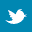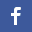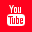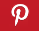Completely Solved C, C++ Programs Assignment.Quick Search Database Videos Tutorials Ebooks Forums FAQ Aboutus Household Industrial Manufacturing Service Shopping Transportation### The Relational Algebra

 Filed Under: DBMS

Relational algebra is a notation for representing the types of operations which can be performed on relational databases. It is used in a RDBMS as the intermediate language for query optimization.
A relation is a set of k-tuples, for some k called the arity of the relation. In general, names are given to the components of the tuple (a tuple corresponds to a record - Pascal or structure - C with fields corresponding to the names of the components). Note: this definition implies that each tuple is unique. Each relation is described by a schema which consists of a relation name and a list of attribute names - relation-name(attribute-list). R(A1, ..., An), R.Ai.
A relational algebra is an algebraic language based on a small number of operators which operate on relations (tables). It is the intermediate language used by a RDBMS. Queries are expressed by applying special operators to relations.

 Figure 1: Relational Algebra expression ::= relation | monadic-expression | dyadic-expression monadic-expression ::= selection | projection | renaming dyadic-expression ::= expression diadic-operation expression selection ::= σselection-condition (relation-name) projection ::= π attribute - list ( relation - name ) renaming ::= ρattribute-list(relation-name) dyadic-operation ::= U| − | × | ∩ | ÷ | |〉〈|join-condition selection-condition ::= logical-condition | comparison

Eight operations were originally defined for relations. Each of these creates a new relation from an existing relation or set of relations.

• Basic Operators
1. Selection: Selects a subset of tuples from a particular relation, based upon a specified selection condition. The selection condition is a boolean expression formed from the names of the attributes of the relation and constants.
2. Projection: Drops columns from a relation retaining only those in the attribute list.
3. Set union: Combines tuples of two relations with like attributes.

• Both relations must have the same number of columns.
• The names of the attributes are the same in both relations.
• Attributes with the same name in both relations have the same domain.

4. Set difference: Finds tuples in two relations with like attributes which are in the first relation but not the second.
5. Cartesian product: Creates a new relation from all concatenations of two relations. NOTE: this is the most computationally expensive operator in the relational algebra.
Renaming: The attribute names in the attribute list replace the attribute names of the relation. ρS(A1,...,An)(R) is the same relation as R but its name is S with the attributes named. A1,...,An.

• Derived operators
1. Set intersection: Finds the common tuples in two relations with like attributes.
2. Divide: Takes two relations, with attributes {X1...XN,Y1...YM} and {Y1...YM} respectively, and returns a relation with attributes {X1...XN} representing all the tuples in the first with matched every tuple in the second relation.
3. Join: Creates new relation from all combinations of tuples in two relations with some matching attributes - R|〉〈|join-conditionS = σjoin-condition (R × S). While this relation has the potential to be computationally expensive (due to the cartesian product) the join-condition typically allows the operation to be relatively inexpensive.

• The join defined above is called a theta-join.
• Equijoins are joins where the join-condition only involves equalities.Back to main directory:DBMS